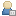###Show Posts

This section allows you to view all posts made by this member. Note that you can only see posts made in areas you currently have access to.

### Messages - mora

Pages: 
1
##### General Discussion / Re: IC from PSAM
« on: May 28, 2019, 04:45:27 pm »
Thanks a lot but I do not know how to read C code.

I am posting an example of a matrix I created using Optimize PSAM and two logos from LogoGenerator. Basically what I want to know if how exactly LogoGenerator translates the PSAM values to nucleotides height in bits and in ddG.

For example: for position 3 G value is 1. How does Logo Generator turns that 1 into a ddD of ~ 3 and ~ 0.6 bits

2
##### General Discussion / Re: IC from PSAM
« on: May 26, 2019, 04:56:22 pm »
Thanks a lot!! One more question:

How exactly logoGenerator calculates the height of each nucleotide at each position?

your Foat et al 2005 paper says that it "the height of each nucleotide is determined by subtracting the smallest weight for any nucleotide at that position and then dividing by the sum of all four weights".

I replicated this on a PSAM I made and the results were similar, but not identical, to the results obtained by Logo nucleotides (y-axis) height.

Does your LogoGenerator makes any other step other than subtracting the smallest weight? maybe some type of normalization?

Ohh I see in your 2006 papers says that for the affinity logo you used the average right? what about when you used the option -style=bits_info? How nucleotide height is calculated then to approximate bits?

3
##### General Discussion / IC from PSAM
« on: May 24, 2019, 04:36:26 pm »
What does the information content (IC) from logos built from a PSAM matrix mean? how does this differ from IC inferred from a classic PSSM/PWM matrix?

Also, is there any information about how LogoGenerator calculate the IC in bits from the values in the PSAM? I assume it has to be very different from how it is calculated according to classic PWM right? (see link)
https://en.wikipedia.org/wiki/Position_weight_matrix

4
##### General Discussion / How do I choose the topology -topo in MatrixREDUCE or MotifREDUCE?
« on: September 13, 2018, 04:23:27 pm »
Is topology equal to kmers size of the binding site? what if I want to explore kmer sizes > 8?

Pages: 
Created and maintained by Dr. Xiang-Jun Lu [律祥俊]. See also http://forum.x3dna.org and http://x3dna.org